Function Repository Resource:

# PolynomialSmithDecomposition

Compute the Smith decomposition of a matrix of univariate polynomials

Contributed by: Daniel Lichtblau
 ResourceFunction["PolynomialSmithDecomposition"][mat] computes the Smith decomposition of the matrix mat of univariate polynomials. ResourceFunction["PolynomialSmithDecomposition"][mat,x] computes the Smith decomposition for a matrix of polynomials in the variable x.

## Details and Options

The result is given in the form {u,s,v}, where u and v are unimodular matrices, s is a diagonal matrix and u.mat.vs. Moreover, each diagonal element of s divides all subsequent diagonal elements.
A unimodular matrix over a ring of univariate polynomials is a matrix with nonzero determinant lying in the coefficient field (that is, constant).
The Smith decomposition is computed using iterations of the Hermite decomposition, as implemented in the resource function PolynomialHermiteDecomposition.
Multivariate polynomials are regarded as univariate in the specified variable, with all others treated as symbolic coefficients.
ResourceFunction["PolynomialSmithDecomposition"] takes Method and Modulus as options. These are the same as for PolynomialHermiteDecomposition.
For specifics of the Method option, see documentation for PolynomialHermiteDecomposition. The only uses in ResourceFunction["PolynomialSmithDecomposition"] are to pass it to the Hermite computations.
ResourceFunction["PolynomialSmithDecomposition"] is intended for matrices of polynomials in a single variable, with all coefficients being exact numbers.

## Examples

### Basic Examples (4)

Compute the Smith decomposition of a 2×3 matrix of low degree polynomials:

 In:=Out=Check the matrix equation:

 In:=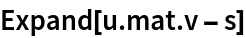Out=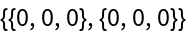Check that u and v are unimodular; that is, they are constants:

 In:=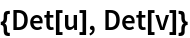Out=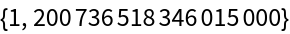Check that the diagonal entries of s divide into subsequent entries:

 In:=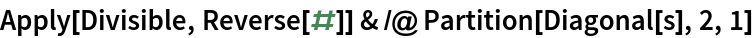Out=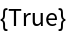### Options (6)

#### Modulus (6)

Generate and compute the Hermite decomposition for an 8×12 matrix of random degree-5 polynomials with coefficients between -10 and 10:

 In:=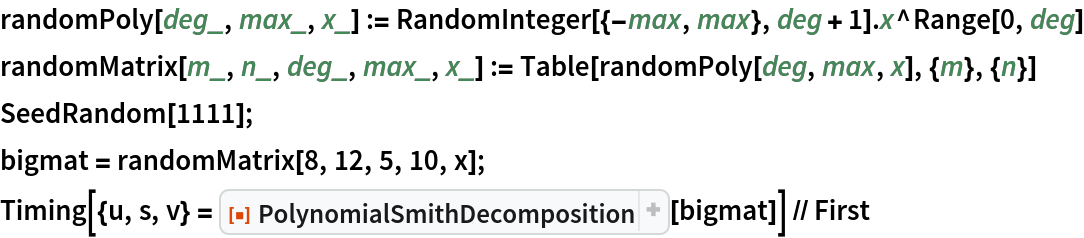Out=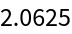As is often the case in symbolic computation, speed improves considerably when one works over a prime field of modest size:

 In:=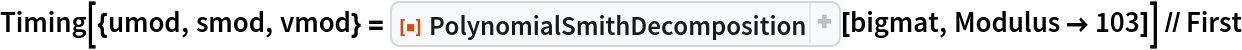Out=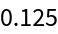Check that the Smith form is a diagonal matrix:

 In:=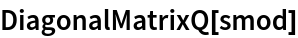Out=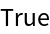Check that the decomposition satisfies the necessary matrix identity:

 In:=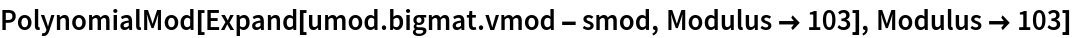Out=Check that the left factor is unimodular:

 In:=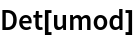Out=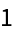The determinant of the right factor cannot easily be computed due to expression swell. A probabilistic test that it is unimodular (that is, a constant) is to check that it gives the same value with multiple substitutions of the polynomial variable:

 In:=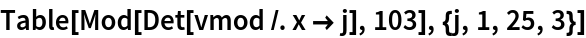Out=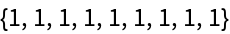### Neat Examples (2)

Use the Smith decomposition to compute the minimal polynomial of a matrix:In:=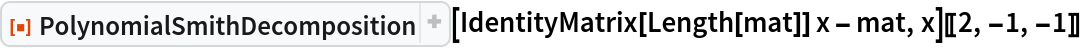Out=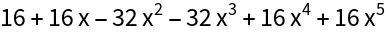The result from PolynomialSmithDecomposition is a scalar multiple of the result of the resource function MatrixMinimalPolynomial:

 In:=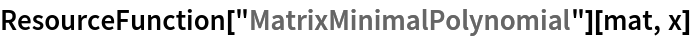Out=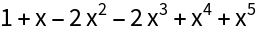For a square matrix mat, the nontrivial diagonal elements in the Smith normal form for mat-x ℑ are the invariant factors of the characteristic polynomial of mat (here ℑ is the identity matrix of same dimension as mat). We can use this to compute the rational canonical form.

We start with code to create a companion matrix from a polynomial:

 In:=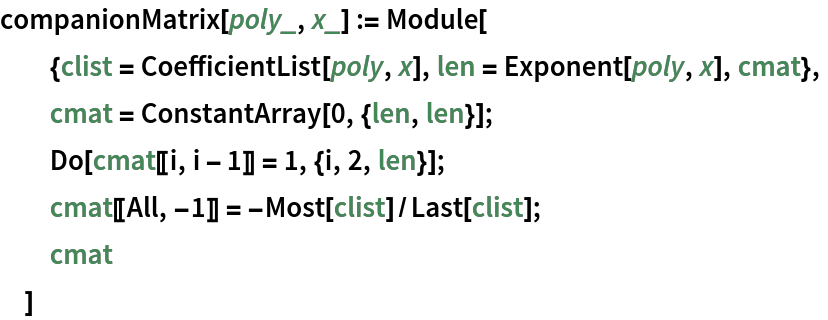Work with an upper triangular matrix having a nontrivial minimal polynomial and hence nontrivial rational canonical form:

 In:=Out=Find the diagonal for the Smith normal form of mat-x:

 In:=Out=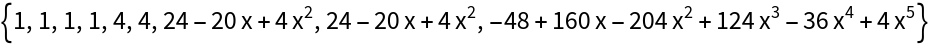Compute the submatrices for the diagonal block:

 In:=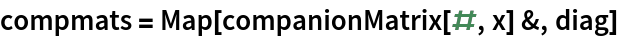Out=Use SparseArray and Band to reconstruct the companion matrix for mat as a diagonal block matrix:

 In:=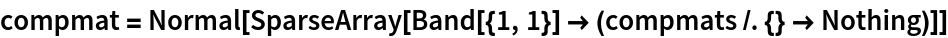Out=## Version History

• 1.0.3 – 18 October 2022
• 1.0.2 – 19 January 2022

## Author Notes

PolynomialSmithDecomposition inherits any weaknesses found in the resource function PolynomialHermiteDecomposition. Since the Smith decomposition uses iterations of the Hermite decomposition, such issues could be compounded. It might not give good results, for example, in the case where input polynomials have approximate coefficients.